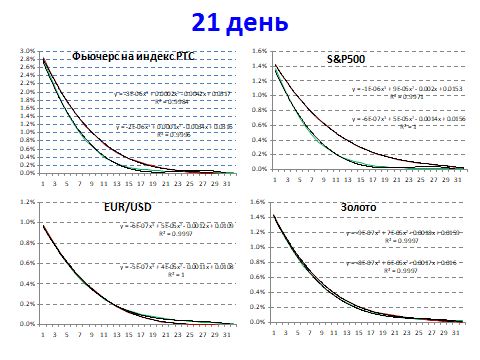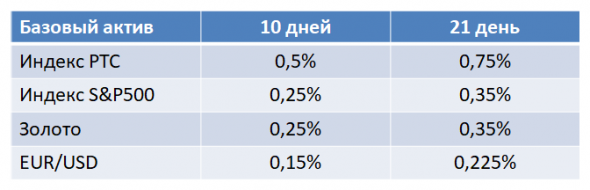# On the grin of volatilityThe topic of options often raises the question of the reasons for the appearance of the “grin of volatility” in options on stock indices. Or, more simply, why out-of-the-money put options with a strike at n% of the current price are more expensive than out-of-the-money calls at the same distance from the current price.

Even smart books are quoted that demand for puts is greater due to the presence of hedgers.

In fact, everything is simpler and different.

Here is a general definition of a “fair” price for an arbitrary payment order.

So, a couple of general definitions.
A payment order is an obligation of the seller to pay a certain amount to the buyer, depending on the price of the underlying asset at a future point in time T – C (T).
The payment function of a payment order is the payment function f (C (T)).

Then the fair price of the payment order can be considered the average f (C (T)) over the distribution of the future price C (T) (most often not known exactly), divided by 1 + R, where R is the risk-free rate until the moment of time T.

What is fair price in the above definition? It means that if the deal is executed at this price, then the average profit of the buyer will be equal to the risk-free rate, and the average loss of the seller will be equal to the risk-free rate. Why is there a risk-free rate with “equity”? It is very simple: the buyer of the payment order, instead of buying, can place funds at a risk-free rate, and the seller of the payment order, having received funds from the sale, can also place these funds at a risk-free rate and, accordingly, on average, lose nothing.

I gave this definition here and showed how, with additional assumptions about the distribution of the future price C (T), one can obtain the classical Black-Scholes formula.

And what is obtained by this formula for “fair” prices, if we simply take the empirical distributions for 2010-2013 as the distribution of the future price C (T)? And here’s what for the periods before expiration of 10 and 21 trading days and a zero risk-free rateThe red line is puts, the green line is calls. The integer value of the X axis is explained by the fact that the coefficient of strike deviation in% of the current price is plotted on it, where the steps in %% are taken in proportion to the standard deviation of the empirical distribution and are equal to:What do we see from the pictures? And the fact that the high cost of puts compared to calls for stock indices is a simple consequence of the properties of price series. At the same time, this should not be the case for the euro-dollar pair, and for gold, the difference is observed at 10 days and much less than for stock indices, and disappears at 21 days.

Hence the question for options connoisseurs: is there a “volatility grin” on the euro-dollar pair, or is there just a “smile”?

If it is not there, then there is no need to “look for a black cat in a dark room” with all sorts of “explanations” about the relative high cost of puts on stock indices due to mythical “hedgers”. The authors of such statements are clearly not the ones to read if you want to understand options.

BUT! The high cost of options really is. My research has shown that, as a rule, option premiums are higher than the “fair” prices shown in the figures. And even adding the rate of short government bonds as “risk-free” does not change it. But the fact of the matter is that this delta (not to be confused with the options delta) is the same for both puts and calls. But everything is clear here: insurance sellers “put” additional risks into prices in comparison with the “average” distribution.##### Fidenge Pecold

My profession is a journalist, but my hobby for 8 years has been studying Forex investing and trading. During this time, I managed to gain extensive experience in investing and trading cryptocurrencies and double my capital in the Forex market. To be the author of this magazine, the site owners invited me to participate in one of the 2020 trading webinars, and I will try to reveal the most relevant crypto market news for you.